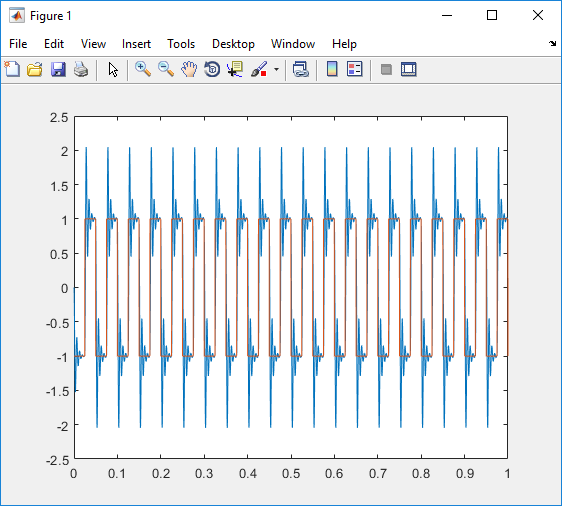## Simulate Simulink Model by Using MATLAB Language

To observe the non-real-time behavior of the model, simulate the Simulink® model .

This procedure uses the Simulink model `slrt_ex_osc_nrt`. To open the model, in the MATLAB Command Window, type:

```open_system(fullfile(matlabroot, 'toolbox', 'slrealtime', ... 'examples', 'slrt_ex_osc_nrt'))```

Before you run the simulation, load this model. From the Modeling tab, click Model Settings. Enable Data Import/Export > Time variable `tout` and Data Import/Export > Output variable `yout`.

1. In the MATLAB Command Window, type:

`output=sim('slrt_ex_osc_nrt','SimulationMode','normal');`

The signal from the signal generator is logged to memory through the Outport block.

2. After Simulink finishes the simulation, type:

`plot(output.get('tout'), output.get('yout'))`

MATLAB® opens a plot window and displays the output response.When you change the model code generation target to `slrealtime.tlc`, build the model, and run the real-time application, the signal data for the `MuxOut` signal is sent to the Simulation Data Inspector. You can export the signal data from the Simulation Data Inspector. For more information, see Inspect and Compare Data Programmatically.